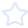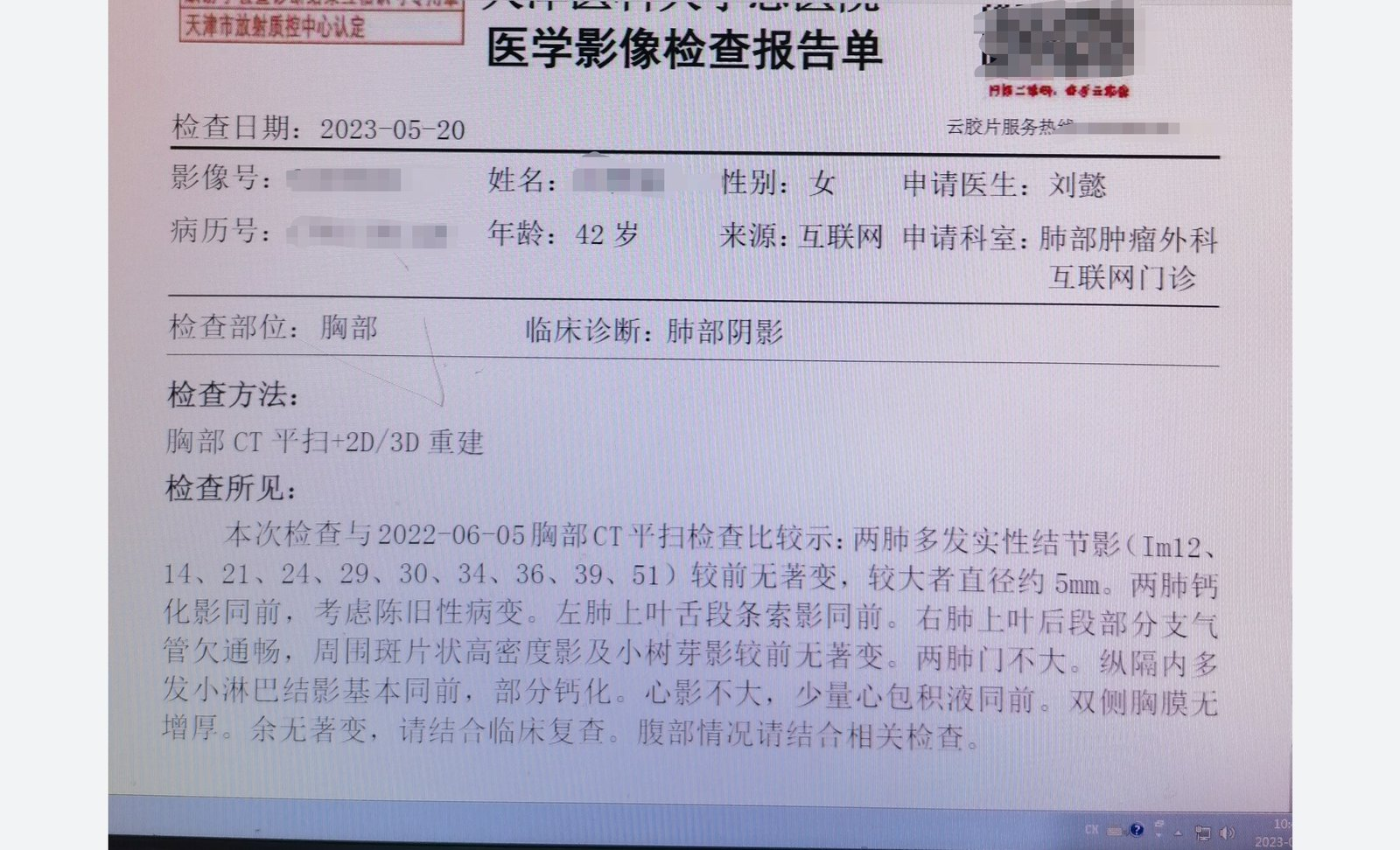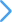'),I.write(o),void((o=I.querySelector("script["+A+'="'+r+'"]'))&&(o.onload=n));var i=I.createElement(f),r=(Object.keys(R.prototype).forEach(function(t){if("src"!==t&&e[t]&&"object"!=typeof e[t])try{i[t]=e[t]}catch(t){}}),i.src=t,i.onload=e.onload,i.onerror=e.onerror,i.setAttribute(A,T()),e.getAttribute("nonce"));r&&i.setAttribute("nonce",r),I.getElementsByTagName("head").appendChild(i)},D=function(e){try{return e.rules}catch(t){try{return e.cssRules}catch(t){return null}}},B=function(e,t,n){var r=I.createElement(a);Object.keys(k.prototype).forEach(function(t){if("href"!==t&&e[t]&&"object"!=typeof e[t])try{r[t]=e[t]}catch(t){}}),r.href=t,r.onload=n||e.onload,r.onerror=e.onerror,r.setAttribute(A,T()),I.getElementsByTagName("head").appendChild(r)},q=function(t){return t?t instanceof N?[t.nodeName,t.src,t.href,t.getAttribute(A)].join(";"):"not_supported":"null"},T=function(){return Math.random().toString(36).slice(2)},L=function(t){return t instanceof R||t instanceof H?t.src:t instanceof k?t.href:""},c={},J=function(t){var e;return Array.isArray(t)?(delete(e=t.reduce(function(t,e,n,r){return t[e]=r[(n+1)%r.length],t},{}))[t[t.length-1]],e):t},X=function(t,e){return t.substr(t.indexOf(e)+e.length,t.length)},Y=function(e,t){return Object.keys(t).filter(function(t){return-1=c[h];if(o&&t instanceof N&&t.hasAttribute(A)&&(i=P(e,l),s(i)),l[r]&&!o){var i=l[r],o=M(e,r,i),r=f(o,e,n);if(null!==r){if("string"!=typeof r)throw new Error("a string should be returned in `onRetry` function");t instanceof R&&!t.getAttribute(j)&&t.src?S(t,r):t instanceof k&&!t.getAttribute(j)&&t.href?B(t,r):t instanceof H&&t.src&&(t.setAttribute(A,T()),t.src=r)}}}}}}}var f=c[m],u=c[p],s=c[v],l=c[b];I.addEventListener("error",a,!0),I.addEventListener("load",function(t){var e,n,r,o,i,c,f;t&&(e=t.target||t.srcElement,!(n=L(e))||e instanceof N&&!e.getAttribute(A)||(r=(c=x(n,l)),o=c,i=P(n,l),c=function(){o&&o[y].push(n),u(i)},e instanceof k?I.styleSheets&&(f=F(I.styleSheets).filter(function(t){return t.href===e.href}),null===(f=D(f))?r&&-1===w.location.href.indexOf(r)&&c():0===f.length?a(t):c()):c()))},!0)}var U={FeHdfImg:["fe1.hdfimg.com","fe2.hdfimg.com"],IHdfImg:["i1.hdfimg.com","i2.hdfimg.com","i3.hdfimg.com","i4.hdfimg.com"],NHdfImg:["n1.hdfimg.com","n2.hdfimg.com","n3.hdfimg.com","n4.hdfimg.com"]};function V(t,e){if("function"==typeof window._seagull_send_log)try{window._seagull_send_log(t,JSON.stringify(e))}catch(t){}}var u=function(t){var e;void 0===t&&(t={});try{if("object"!=typeof t[b])throw new Error("opts.domain cannot be non-object.");var n=[h,m,p,v,b],r=Object.keys(t).filter(function(t){return-1===n.indexOf(t)});if(05届年度好今晚福彩3D开奖结果收藏

# 刘懿博士说肺癌（五八三二）42岁女士有十多个肺结节，心理压力有点大×